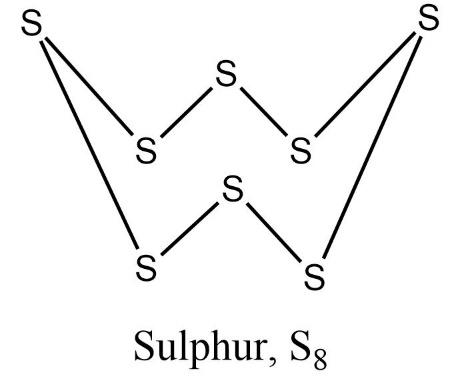QuestionAnswers

# Molecular formula of oxygen is ${{O}_{2}}$ while sulphur is ${{S}_{8}}$, why ?

Hint: Write the electronic configuration of oxygen and sulphur. Think about what happens to atomic size when you go down the group. Also, think about physical states of oxygen and sulphur.

- Oxygen and sulphur belongs to the Group 16 family of chalcogens. Oxygen has the electronic configuration as follows,
${}^{8}O=1{{s}^{2}}2{{s}^{2}}2{{p}^{4}}$
- Oxygen is a gas while all other chalcogens are solids at room temperature.
- Oxygen has the potential to form a $p\pi -p\pi$ bond. This $p\pi -p\pi$ overlapping between two oxygen atoms leads to the formation of a diatomic stable ${{O}_{2}}$ molecule having a linear structure (O=O). Moreover, these ${{O}_{2}}$ molecules have only weak Van der Waals’ forces of interaction between them and so it exists in a gaseous state.
- Now, let’s have a look at sulphur.
The electronic configuration of sulphur is,
${{S}_{2}}=1{{s}^{2}}2{{s}^{2}}2{{p}^{6}}3{{s}^{2}}3{{p}^{4}}$
- As we move down the group, atomic size increases due to addition of a greater number of orbitals. Due to the large size of sulphur and also the presence of vacant 3d orbitals, sulphur is not able to form $p\pi -p\pi$ bonds.
- The p-orbitals of adjacent sulphur overlap axially only giving rise to $\text{ }\!\!\sigma\!\!\text{ -bonds}$. But one atom of sulphur needs to form at least two such bonds to attain noble gas configuration. Similarly, each sulphur needs to form a stable configuration. This can only be achieved by formation of a cyclic ring.
So, eight sulphur atoms join together to form a polyatomic crown shaped puckered ring.Therefore, we can justify that, the molecular formula of oxygen is ${{O}_{2}}$ while sulphur is ${{S}_{8}}$.

Note: Like sulphur, selenium also forms a puckered ring structure. Also, polyatomic molecules have strong Van der Waals’ forces of interaction between them and so they exist as solids at room temperature. Remember atomic radii increases down the group and decreases as we move from left to right in the periodic table.